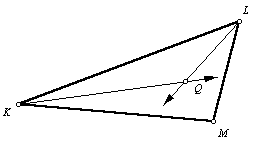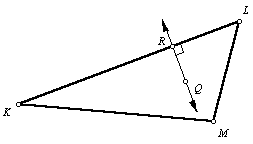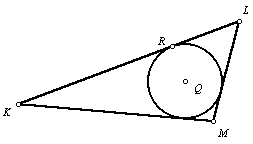Given a triangle, inscribe a circle.

 1. Begin with triangle KLM.2. Construct the bisectors of Ð K and Ð L. (Refer to the angle bisector construction.) Let point Q be the intersection of the two angle bisectors.3. Construct a line through point Q, perpendicular to line segment KL. Let point R be the point of intersection. (Refer to the construction of a perpendicular line through a given point.)4. Center the compass on point Q, and draw a circle through point R. The circle will be tangent to all three sides of a triangle.Back to Geometry Construction Reference